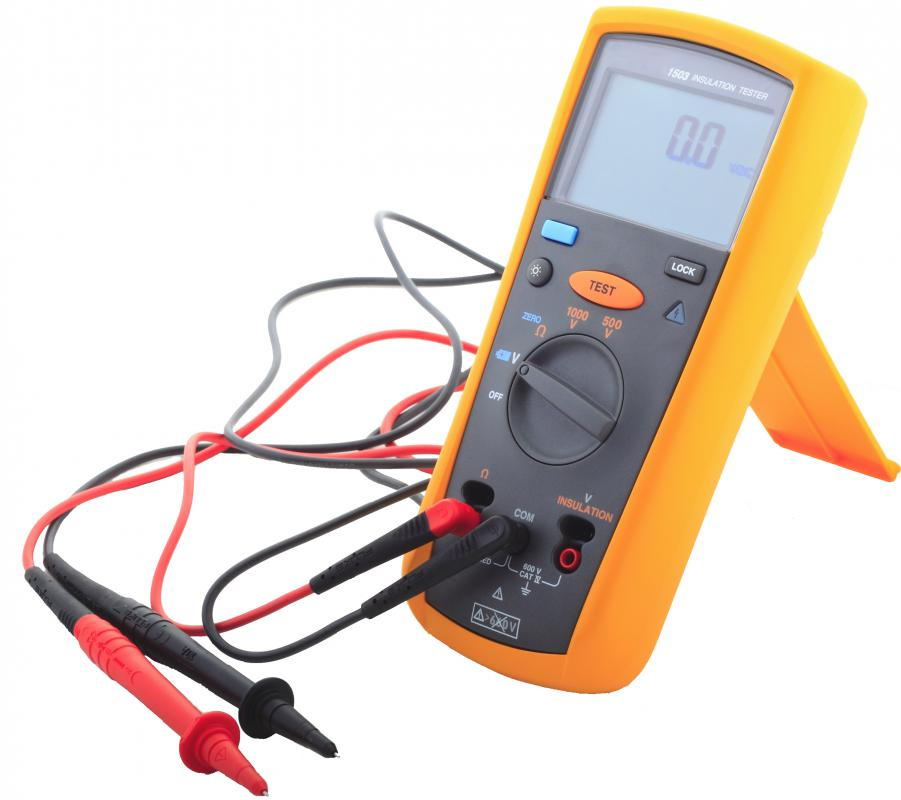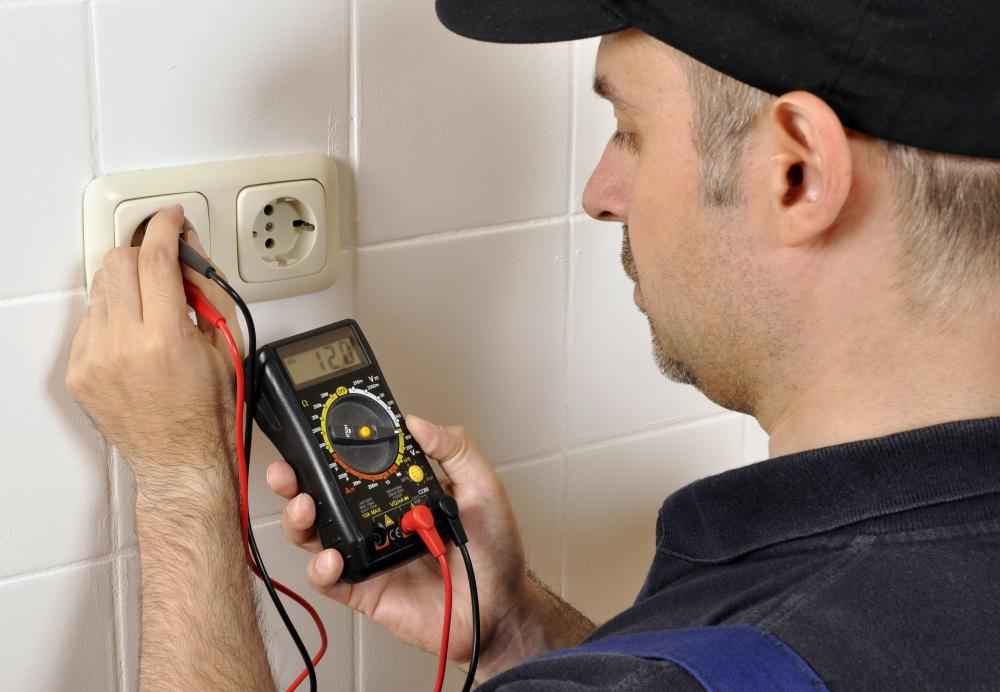# What are Electrical Measurements?

CW Deziel

Electrical measurements are those that measure the voltage, current, and power and resistance in an electric circuit. Also included are those that measure electrical characteristics of the circuit itself and the materials or components comprising it. In addition, electrical measurements are measurements made of the electromagnetic field surrounding a conductor carrying an electric current. All types of electrical measurements involve methods, devices, and calculations specific to the measurements being made.A digital multimeter is a tool that can measure amps, volts, and ohms.

The most commonly-measured quantities in an electrical circuit are usually determined with a meter. An ammeter measures current in amperes, a voltmeter measures voltage in volts, and an ohmmeter measures resistance in ohms. The electrical power of a circuit in watts, which is the product of its voltage and current, can be calculated once those two quantities have been determined, or, if the resistance and one of the quantities is known, by applying Ohm’s Law. The functions of ammeter, voltmeter, and ohmmeter are combined in a device known as a multimeter. It has a switch on the front that allows the user to select the function of the meter and the sensitivity of measurement.Voltmeters are used to measure the voltage traveling between two points within a circuit.

The electrical field around a conductor can affect other conductors in its vicinity, and electrical measurements of its characteristics can often be deduced from the effect it has on these conductors. If the electric current in a conductor is changing, or is in flux, it generates a magnetic field capable of inducing a current in any other conductor within the field. The magnetic field around a conductor with a changing electric current, such as one carrying an alternating current in a constant state of flux, can be measured with a Hall sensor. A stationary current, on the other hand, generates an electrostatic field that can be determined with an electrometer, which measures the force of repulsion induced by the field in two similar conductors.

Electrical circuits and their components have characteristics that affect the ability of the circuit to conduct a current and generate a magnetic field. Electrical measurements of these characteristics are often determined by calculations based on measurable quantities of the circuit, like voltage, current, and resistance. For example, the capacitance of an electrical device designed to hold a charge, like a battery, is determined from measurements of the electrical power and time taken to charge it. Inductance, the ability of a circuit to generate a voltage when immersed in a magnetic field, can be inferred by measuring the strength of the field with a Hall sensor and the amount of current generated in the circuit with an ammeter.